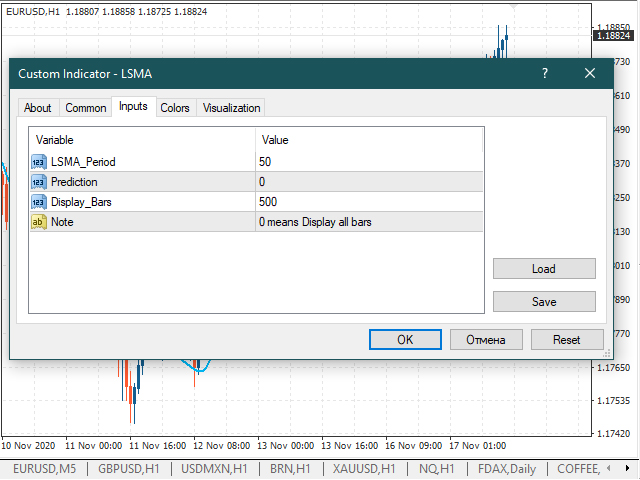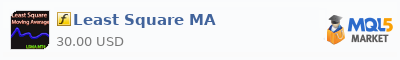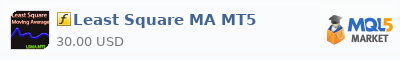# Least squares moving average indicator (LSMA)

Content:

## Indicator history

Unfortunately, there is no mention on the Internet who was the first to apply the least squares method for stock prices. The first mentions appear only after the 2000s, so we can say that this indicator is “new” and not fully explored. This has become possible thanks to the increase in computing power. If you have more accurate information, share with us in the comments, we will supplement the section.

## Description and input parameters

The Least Square Moving Average indicator allows you to assess the direction of the market movement and its possible reversals. The indicator is a line that is displayed in the price window. The least squares moving average algorithm plots a regression line on the graph at the current point. That is, the LSMA tries to predict the price behavior, what it would be if the regression line continued.
Input parameters:LSMA_Period – period for calculating the moving average by the least squares method;

Prediction – the number of candles used to construct the predicted moving average by the least squares method, provided that the rate of its (decline or growth remains);

Display_Bars – number of displayed bars (at 0, all are displayed).

The first difference is that LSMA is a mathematical expected line and is related to price through a formula. Therefore, it can show values that prices have never shown.The second is that the least squares moving average is much more responsive than any other moving average. Given that its calculation accuracy will be higher. The figure shows an exponential moving average with parameters 50 and 15 and LSMA with a value of 50.An interesting feature of this indicator is the Prediction parameter. It draws a line, which would be if the price moved at the same speed for a given number of candles. In fact, we can determine the points at which the price stopped. To do this, add a least squares moving average with period n (Prediction = 0) and exactly the same one with parameter n and Prediction not equal to 0. It follows from the formula that if the speed is 0, then the LSMA line will coincide with the LSMA + Prediction line.## Calculation formula

The indicator is based on the least squares method to find the straight line that best matches the data for the selected period. Therefore, we write the equation of the straight line:

y=ax+b;

Because we are looking for the y-value at the current point (x = 0), so we need the coefficient b.
The coefficient a and b is found from the system of equations:Where xi is the ordinal number of the candlestick from right to left, yi is the price value corresponding to the candle, n is the quantity candles (period).
Consider an example: We have 5 candles

 1 2 3 4 5 42,005 40,675 39,76 41,215 41,63

For the sake of a more compact notation, the “counter” variable can be omitted, since it is clear that the summation is carried out from 1 to n = 5.

 xi 1 2 3 4 5 Σxi 15 yi 42,005 40,675 39,76 41,215 41,63 Σyi 205,285 xi2 1 4 9 16 25 Σxi2 55 xiyi 42,005 81,35 119,28 164,86 208,15 Σxiyi 615,645

Substituting the numbers into the system and performing the calculations, we get the value b = 41.12. In this case, the value of the usual moving average will be 41.057. For period 5, we already see a small difference. At the same time, the LSMA price is closer to the last price (42.005) than the usual moving average.

## Examples of using

One of the signals is the leading of the moving average according to the method of least squares of the maximum or minimum price. Example of a sell signal:Example for a buy and sell signal:An example using two LSMA (50) with Prediction = 5 and Prediction = 0.Of course, all strategies for crossing two or three moving averages and many others can be used with the Least Square MA indicator. Thus, improving its response and adaptability to changes in its strategy.